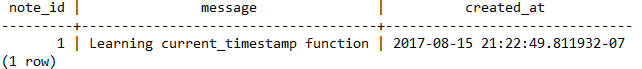# PostgreSQL Tutorial: CURRENT_TIMESTAMP Function

September 17, 2023

The PostgreSQL `CURRENT_TIMESTAMP()` function returns the current date and time with time zone, which is the time when the transaction starts.

## Syntax

The following illustrates the syntax of the PostgreSQL `CURRENT_TIMESTAMP()` function:

``````CURRENT_TIMESTAMP(precision)
``````

## Arguments

The PostgreSQL `CURRENT_TIMESTAMP()` function accepts one optional argument.

1) `precision`

The `precision` specifies the number of digits in the fractional seconds precision in the second field of the result.

If you omit the `precision` argument, the `CURRENT_TIMESTAMP()` function will return a `TIMESTAMP` with a time zone that includes the full fractional seconds precision available.

## Return value

The `CURRENT_TIMESTAMP()` function returns a `TIMESTAMP WITH TIME ZONE` that represents the date and time at which the transaction started.

## Examples

The following example shows how to use the `CURRENT_TIMESTAMP()` function to get the current date and time:

``````SELECT CURRENT_TIMESTAMP;
``````

The result is:

``````              now
-------------------------------
2017-08-15 21:05:15.723336+07
(1 row)
``````

Internally, the `CURRENT_TIMESTAMP()` is implemented with the `NOW()` function, therefore, the column alias is `NOW`.

Like the `NOW()` function, the `CURRENT_TIMESTAMP()` function can be used as the default value of a timestamp column.

Let’s take a look at the following example.

First, create a table named `note` that has the `created_at` column is a `TIMESTAMP WITH TIME ZONE` column.

``````CREATE TABLE note(
note_id serial PRIMARY KEY,
message varchar(255) NOT NULL,
created_at TIMESTAMP WITH TIME ZONE DEFAULT CURRENT_TIMESTAMP
);
``````

The default value of the `created_at` column is provided by the result of the `CURRENT_TIMESTAMP()` function.

Second, insert a new row into the `note` table:

``````INSERT INTO note(message)
VALUES('Testing current_timestamp function');
``````

In this statement, we did not specify the value for the `created_at` column, therefore, it defaulted to the timestamp at which the transaction started.

Third, verify whether the insert has been taken place correctly using the following query:

``````SELECT
*
FROM
note;
``````

The following picture illustrates the result:As you can see the `created_at` column was populated by the date and time at which the statement executed.

## Remarks

In PostgreSQL, the `TRANSACTION_TIMESTAMP()` function is equivalent to the `CURRENT_TIMESTAMP` function. However, the function name `TRANSACTION_TIMESTAMP` clearly reflects what the function returns.

In this tutorial, you have learned how to use the PostgresQL `CURRENT_TIME()` to get the date and time at which the transaction starts.